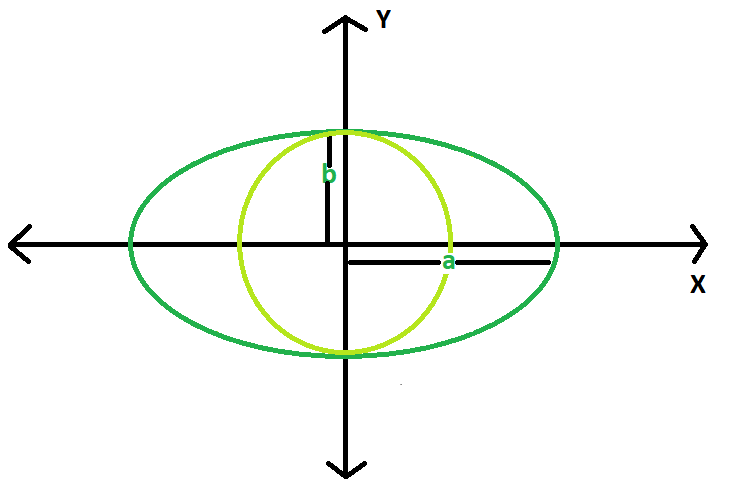Open In App

# Find the area of largest circle inscribed in ellipse

Given an ellipse, with major and minor axis length 2a & 2b respectively. The task is to find the area of the largest circle that can be inscribed in it.
Examples:

```Input : a = 5, b = 3
Output : 28.2743

Input : a = 10, b = 8
Output : 201.062```Approach : The maximal radius of the circle inscribed in the ellipse is the minor axis of the ellipse.
So, area of the largest circle = ? * b * b.
Below is the implementation of the above approach:

## C++

 `// CPP program to find``// the area of the circle``#include ``using` `namespace` `std;``#define pi 3.1415926` `double` `areaCircle(``double` `b)``{``    ``double` `area = pi * b * b;``    ``return` `area;``}` `// Driver Code``int` `main()``{``    ``double` `a = 10, b = 8;``    ``cout << areaCircle(b);``    ``return` `0;``}`

## Java

 `// Java Program to find the area``// of circle` `class` `GFG``{``    ``static` `double` `areaCircle(``double` `b)``    ``{``    ` `    ` `        ``// Area of the Reuleaux triangle``        ``double` `area = (``double``)``3.1415926` `* b * b;``        ``return` `area;``    ``}``    ` `    ``// Driver code``    ``public` `static` `void` `main(String args[])``    ``{``        ``float` `a = ``10``,b = ``8``;``        ``System.out.println(areaCircle(b)) ;``    ``}``}` `// This code is contributed by mohit kumar 29`

## Python3

 `# Python3 program implementation of above approach` `import` `math` `# Function to return required answer``def` `areaCircle(b):``    ``area ``=` `math.pi ``*` `b ``*` `b``    ``return` `area`  `# Driver Code``a ``=` `10``b ``=` `8``print``(areaCircle(b))` `# This code is contributed by``# Sanjit_Prasad`

## C#

 `// C# Program to find the area``// of circle``using` `System;` `class` `GFG``{``    ``static` `double` `areaCircle(``double` `b)``    ``{``        ``// Area of the Reuleaux triangle``        ``double` `area = (``double``)3.1415926 * b * b;``        ``return` `area;``    ``}``    ` `    ``// Driver code``    ``public` `static` `void` `Main()``    ``{``        ``float` `b = 8;``        ``Console.WriteLine(areaCircle(b)) ;``    ``}``}` `// This code is contributed by aishwarya.27`

## PHP

 ``

## Javascript

 ``

Output:

`201.062`

Time Complexity: O(1)

Auxiliary Space: O(1)